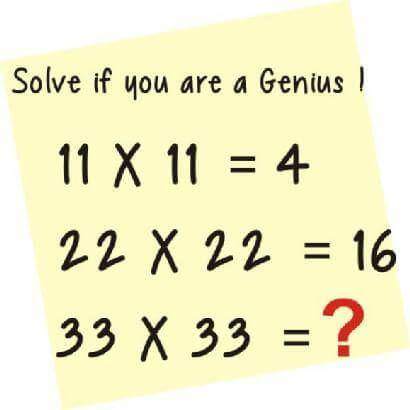### Are You a Genius? Solve This

Most people will fail to give the correct answer to this question. If you can solve this, then you are truly a Genius.#### Comments (80)

27
Follow
The answer is 18
Follow
36
Follow
11×11=2×2=4
22×22=4×4=16
33×33=6×6=36
Follow
The answer is 64
using geometric progression
T1 = 4
T2 = 4^2 = 16
T3 = 4^3 = 64
Follow
256
Follow
thus 11*11=4 and 22*22=16 then 33*33=36
Follow
36
Follow
64 is the answer. 4 is 4^1. 16 is 4^2. So the third one should be 4^3, which is 64
Follow
256
Follow
28
Follow
(3+3) × (3+3)
= 36
Follow
D Answer Is 81 :33*33=(3*3)=9*(3*3)=9;(9*9)=81
Follow
the ans is 36 dont av tym to explain
Follow
No ypu can't dear,to study biology,you need chemistry,biology,maths,english,and agricultural science and any other subject
Follow

#### Quick Questions

• 3 Answers   ·   5 hours ago
• 3 Answers   ·   2 hours ago
• 4 Answers   ·   10 hours ago
To get notifications when anyone comments,

#### Post Comment

Please don't post or ask to join a "Group" or "Whatsapp Group" as a comment. It will be deleted. To join or start a group, please click here

1 Members
• 29th
Aug 2019
• 30th
Aug 2019
##### Marketplace
• Here are the things students around you have to sell;
• ###### beads & Wirer Works
₦20,000   New
• ###### Nice and beautiful polos
₦2,000   UNIZIK   New